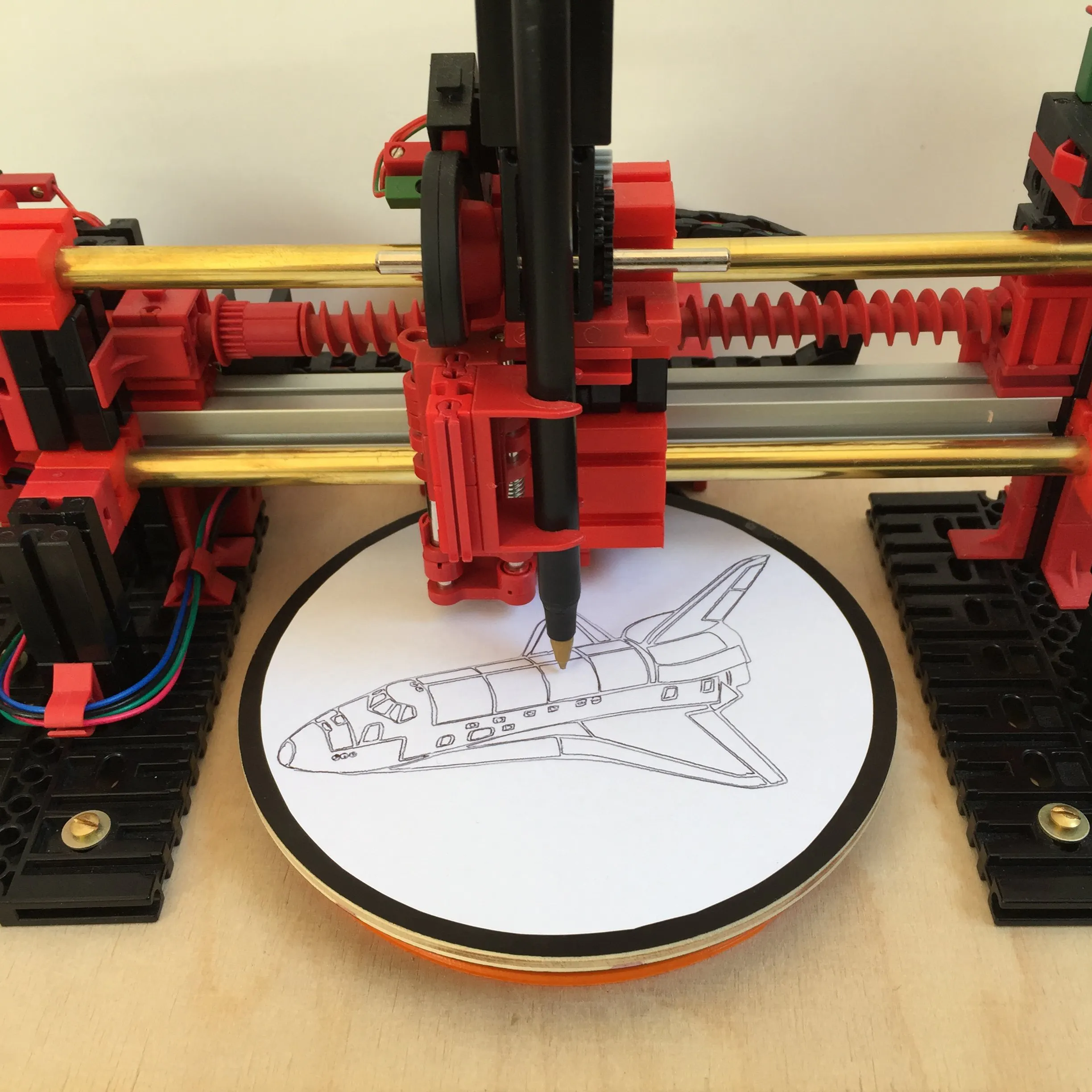# Polar Drawing Machine

Polar drawing machine built with fischertechnik and Arduino. Thanks to a G-code interpreter, it can draw any bitmap or vector graphics.

## Things used in this project

### Software apps and online servicesArduino IDEMicrosoft Visual Studio 2015

## Custom parts and enclosures

### Gear

180 teeth gear for timing belt

## Code

### Plotter Firmaware 3.1

Arduino
```#include <MegaDueShield.h>

#define BUFFER_SIZE 1200
#define RAXIS_ZERO 2822 // Kugelschreiber

/*  Plotter Firmware 3_1

March, 2017

requires a G-code preprocessor
requires C# App to load G-code

G-code is transferred while drawing
optimized parallelization of serial communication and drawing
optimized serial communication

*/

StepperMotor & nema14 = *shield.getStepper(1);
StepperMotor & nema17 = *shield.getStepper(2);

DCMotor & stift = *shield.getDCMotor(1);

long posn14 = 0;
long posn17 = 0;

uint8_t lastCommand = 0;
boolean complete = false;
uint8_t commands[BUFFER_SIZE];
int commandPos[BUFFER_SIZE];

/*  G-Code commands:
G00: Rapid positioning
G01: Linear interpolation
G02: Circular interpolation, clockwise
G03: Circular interpolation, counterclockwise

M03: Spindle on: Pen down
M05: Spindle off: Pen up
*/

/* 11 Umdrehungen = 52,3mm
2200 Schritte = 52,3mm
1mm = 41,35338 Schritte

r = 0mm: 2843 Schritte
pos (r) = 2843 - r * 41,35338
*/

long rToPos(float r)
{
return RAXIS_ZERO - (r * 41.885);
}

int rStepsToPos(int steps)
{
return RAXIS_ZERO - steps;
}

/* 2Pi = 1800 Schritte

pos (phi) = phi / 2Pi * 1800
*/

long phiToPos(float phi)
{
while (posn17 >= 1800)
{
posn17 -= 1800;
}
while (posn17 < 0)
{
posn17 += 1800;
}
float posf = phi / 2.0 / PI * 1800;
long pos = (long) posf;

if (pos - posn17 > 900)
{
pos -= 1800;
}

if (posn17 - pos > 900)
{
pos += 1800;
}
return pos;
}

int phiStepsToPos(int steps)
{
while (posn17 >= 1800)
{
posn17 -= 1800;
}
while (posn17 < 0)
{
posn17 += 1800;
}
if (steps - posn17 > 900)
{
steps -= 1800;
}

if (posn17 - steps > 900)
{
steps += 1800;
}
return steps;
}

void homeR()
{
int counter = 0;
{
long pos = posn14 - 1;
bool t = false;
while (!t) {
t = nema14.stepping(posn14, pos);
}
counter++;
}
posn14 = 0;
//Serial.println("Referenzpunkt");
//Serial.println(counter);
}

void penZ(bool value)
{

if (value)
{
// Pen up
{
stift.ccw(200);
}
stift.stop();
}
else
{
// Pen down
stift.cw(200);
delay(500);
stift.stop();
}
}

bool findCharacter(char character)
{
if (Serial.available() > 0)
{
if (serialCharacter == character)
{
return true;
}
}
return false;
}

int parseInteger()
{
bool delimiter = false;
int returnInt = 0;
while (!delimiter)
{
while(Serial.available() < 1) {}
character -= 48;
if(character >= 0 && character <= 9)
{
returnInt *= 10;
returnInt += character;
}
else
{
delimiter = true;
}
}
return returnInt;
}

bool readGCodeLine(uint8_t & command, int arguments[])
{
//int arguments;
Serial.print('n');
Serial.print('\n');
Serial.flush();
while (!findCharacter('G')) {}
command = parseInteger();
switch (command) {
case 0:
while (!findCharacter('P')) {}
arguments = parseInteger();
while (!findCharacter('R')) {}
arguments = parseInteger();
break;
case 8:
complete = true;
break;
case 9:
while (!findCharacter('Z')) {}
arguments = parseInteger();
break;
}
}

bool stepwiseReadGCodeLine(uint8_t & milestone, uint8_t & command, int arguments[])
{
switch (milestone)
{
case 0:
Serial.print('n');
Serial.print('\n');
Serial.flush();
milestone++;
return false;
case 1:
if (Serial.available() > 0) {
milestone++;
}
return false;
case 2:
if (findCharacter('G'))
{
milestone++;
}
return false;
case 3:
command = parseInteger();
milestone++;
return false;
case 4:
switch (command)
{
case 0:
milestone = 5;
break;
case 8:
complete = true;
milestone = 8;
break;
case 9:
milestone = 7;
break;
}
return false;
case 5:
if (findCharacter('P'))
{
arguments = parseInteger();
milestone++;
}
return false;
case 6:
if (findCharacter('R'))
{
arguments = parseInteger();
milestone = 8;
}
return false;
case 7:
if (findCharacter('Z'))
{
arguments = parseInteger();
milestone = 8;
}
return false;
case 8:
return true;
}
}

void processGCode()
{
uint8_t command;
int arguments;
int nextArguments;
uint8_t milestone = 0;

while(!findCharacter('s')) {}

readGCodeLine(command, arguments);  // get first G-code line
nema14.wakeUp();
delay(10);
while (!complete)
{
bool t = false;
milestone = 0;
switch (command)
{
case 0:
while (!t) {
t = nema14.stepping(posn14, rStepsToPos(arguments));
t &= nema17.stepping(posn17, phiStepsToPos(arguments));
}
arguments = nextArguments;
arguments = nextArguments;
break;
case 9:
penZ(arguments);
break;
case 8:
break;
}
}
}

void setup() {
Serial.begin(115200);

delay(1000);
nema14.motorConfig(200, 190, 600, 400);
nema17.motorConfig(200, 120, 280, 400);

// Stift
pinMode(C4, INPUT_PULLUP);
penZ(1);

// R-Achse
pinMode(D4, INPUT_PULLUP);
homeR();
while (!nema17.stepping(posn17, 900)) {}
posn17 = 0;
nema14.release();

delay(400);

//fahren();
complete = false;
processGCode();
int p = posn17 + 900;
bool t = false;
while (!t)
{
t = nema17.stepping(posn17, p);
t &= nema14.stepping(posn14, 50);
}
nema14.release();
}

void loop() {
// put your main code here, to run repeatedly:

}
```

## Credits

### David

4 projects • 110 followers
Engineering student